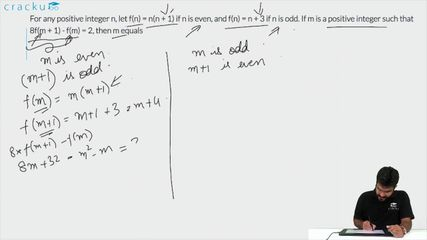Question 78

# For any positive integer n, let f(n) = n(n + 1) if n is even, and f(n) = n + 3 if n is odd. If m is a positive integer such that 8f(m + 1) - f(m) = 2, then m equals

Solution

Assuming m is even, then 8f(m+1)-f(m)=2

m+1 will be odd

So, 8(m+1+3)-m(m+1)=2

=> 8m+32-$$m^2-m$$=2

=> $$m^2-7m-30=0$$

=> m=10,-3

Rejecting the negative value, we get m=10

Assuming m is odd, m+1 will be even.

then, 8(m+1)(m+2)-m-3=2

=> 8($$m^2+3m+2$$)-m-3=2

=> $$8m^2+23m+11=0$$

Solving this, m = -2.26 and -0.60

Hence, the value of m is not integral. Hence this case will be rejected.

### View Video Solution